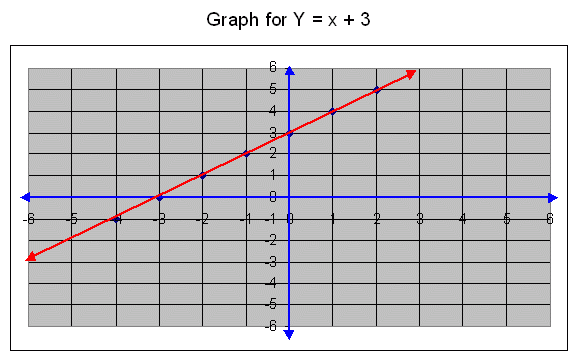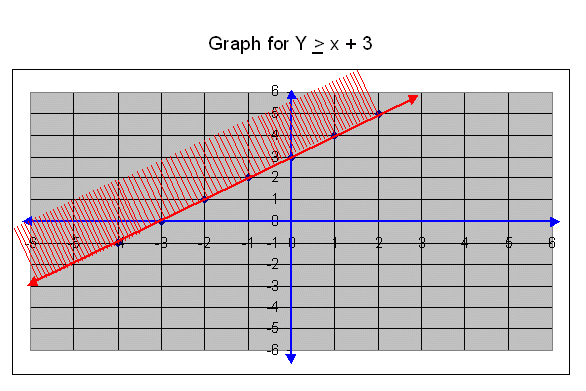Email us to get an instant 20% discount on highly effective K-12 Math & English kwizNET Programs!

#### Online Quiz (WorksheetABCD)

Questions Per Quiz = 2 4 6 8 10

### Middle/High School Algebra, Geometry, and Statistics (AGS)2.2 Graph for y ł x + 3

Draw a graph for y ł x + 3.

First we draw a straight line for y = x + 3.

The ordered pairs for the graph y = x+3.
x-4-3-2-1012
y = x + 3-1012345
Ordered pairs(-4,-1)(-3,0)(-2,1)(-1,2)(0,3)(1,4)(2,5)To find out the region for y ł x + 3, first we find out that the orgin lies in the region represented by the graph or not.
We substitute the coordinates of the origin (0,0) in the given inequation,
y ł x + 3, we get,
0 ł 0 + 3
0 ł 3, this is wrong.
The origin doew not lie in the half plane represented the graph.
Graph for y ł x + 3, isThe boundary line y = x + 3 lies in the region represented by above graph. The boundary line is drawn as a dark line. The region indicated by the graph is shaded.

Its solution set is {(x,y)/(x,y) Î R and y ł x + 3}

Directions: Draw the graphs for given conditions.

1. Draw a graph for y ł x + 6.

2. Draw a graph for y ł x + 1.

3. Draw a graph for y ł x + 2.

4. Draw a graph for y ł x + 4.

5. Draw a graph for y ł x + 5.